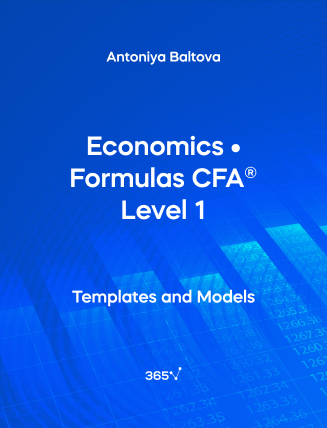## Economics • Formulas CFA® Level 1###### Antoniya Baltova

Need an all-in-one list with the Economics formulas included in the CFA® Level 1 Exam? We have compiled them for you here. The relevant formulas have been organized and presented by chapter. In this section, we will cover the following topics — Demand and Supply Analysis, Firm and Market Structures, Aggregate Output, Prices, and Economic Growth, Business Cycles, Monetary and Fiscal Policy, International Trade, Capital Flows, and Currency Exchange Rates.

## 1. Topics in Demand and Supply Analysis

Price Elasticity

Price~elasticity = \frac {\%Δ~Quantity~demanded~(Qx)}{\%Δ~Price~(Px)}

0 > e > -1 rightarrow inelastic demand
-1 > e > -∞ rightarrow elastic demand
e = -1 rightarrow unit elastic demand
e = 0 rightarrow perfectly inelastic demand
e = -∞ rightarrow perfectly elastic demand

Income Elasticity

Income~elasticity = \frac {\%Δ~Quantity~demanded~(Qx)}{\%Δ~Income~(Ix)}

e > 0 rightarrow normal goods
e < 0 rightarrow inferior goods
ε{_Y} = Income elasticity

Cross-price Elasticity

Cross-price~elasticity = \frac {\%Δ~Quantity~demanded~(Qx)}{\%Δ~Price~of~a~related~good~(Py)}

e > 0 rightarrow the related product is a substitute
e < 0 rightarrow the related product is a complement
y = Related product
ε{_{py}} = Cross-price elasticity

## 2. The Firm and Market Structures

For all market structures, Max Profit \longrightarrow when MC = MR

MC = Marginal cost
MR = Marginal revenue

Breakeven points:
AR = ATC (perfect competition)
TR = TC (imperfect competition)

ATC = Average Total Cost
AR = Average Revenue
TR = Total Revenue
TC = Total Cost
AR = ATC holds true in imperfect competition

Short-run shutdown points:
AR < AVC (perfect competition)
TR < TVC (imperfect competition)

Market structures:
Perfect Competition
Monopolistic Competition
Oligopoly
Monopoly

## 3. Aggregate Output, Prices, and Economic Growth

Total GDP = Final value of goods and services produced (market value)
+ Government services (at cost)
+ Rental value of owner-occupied housing (an estimate)

GDP~Deflator =  \frac{Nominal~GDP}{Real~GDP} \times 100
Nominal~GDP{_t} = P{_t} \times Q{_t}
Real~GDP{_t} = P{_{b}} \times Q{_t}

t = Current year
b = Base year
P{_t} = Prices in year {_t}
P{_b} = Prices in base year
Q{_t} = Quantity produced in year {_t}

Expenditure Approach

Real~GDP = Consumption~spending~(C) + Investment~(I) + Government~spending~(G) + Net~exports~(X-M)

X = Exports
M = Imports

Income Approach

Real~GDP = National~income + Capital~consumption~allowance + Statistical~discrepancy
Real~GDP = Consumption~spending~(C) + Savings~(S) + Taxes~(T)
Savings~(S) = Investments~(I) + Fiscal~Balance~(G-T) + Trade~Balance~(X-M)
S – I = Fiscal~Balance~(G-T) + Trade~Balance~(X-M)

National Income = Employees’ compensation
+ Corporate and government profits before taxes
+ Interest income
+ Rent
− Subsidies

Personal Income = National income
+ Transfer payments (social insurance, unemployment or disability payments)
− Corporate income taxes
− Undistributed corporate profits

Personal Disposable Income = Personal income – Personal taxes

Potential GDP = Aggregate hours worked × Labor productivity
\longrightarrow Aggregate hours worked = Labor force × Average hours worked per week
\longrightarrow Growth in Potential GDP = Growth in labor force + Growth in labor productivity

The Production Function

Y = A \times f (K, L)

Y = Aggregate output
A = Total Factor Productivity (TFP)
K = Capital
L = Labor

Growth in Potential GDP = Growth in technology + WL × (growth in labor) + WC × (growth in capital)
WL = Labor’s percentage share of national income
WC = Capital’s percentage share of national income

Unemployment~Rate = \frac {Number~of~unemployed~people}{Total~labor~force}
Participation~Rate~(Activity~Ratio) = \frac {Total~labor~force}{Total~working–age~population}
Labor~Force = Unemployed~people + Employed~people

Unemployed = Looking for job

Consumer~Price~Index = \frac {Cost~of~basket~at~current–year~prices}{Cost~of~basket~at~base–year~prices} \times 100
Laspeyres’ Index = \frac {\Sigma~(Current–year~price \times Base–year~quantity)}{\Sigma~(Base–year~price \times Base–year~quantity)}
Fisher’s~Index = \sqrt {(Laspeyres’~Index) \times (Paashe~Price~Index)}
Paashe~Price~Index = \frac {\Sigma~(Current–year~price \times Current–year~quantity)}{\Sigma~(Base–year~price \times Base–year~quantity)}

## 4. Monetary and Fiscal Policy

Money~Multiplier = \frac {1}{Reserve~requirement}
Fiscal~Multiplier = \frac {1}{1- MPC \times (1- t)}

MPC = Marginal propensity to consume
t = Tax rate

Equation of Exchange

MV = PY~(Money~supply \times Velocity = Price \times Real~output)

Fisher Effect

Nominal~Interest~Rate = Real~interest~rate + Expected~inflation~rate

Neutral Interest Rate

Neutral~interest~rate = Real~trend~rate~of~economic~growth + Inflation~target

## 5. International Trade and Capital Flows

GDP

GDP = C + I + G + X - M

C = Consumption
I = Investments
G = Government Spending
X = Export
M = Import

Balance of Payments

Current~Account + Capital~Account + Financial~ Account = 0

X - M = Private~Savings + Government~Savings - Investments~in~domestic~capital
Real~Exchange~Rate = Nominal~exchange~rate \times \frac {CPI~base~currency}{CPI~price~currency}